## Monday, September 30, 2019

### Hearsay

(Click on the comic if you can't see the full image.)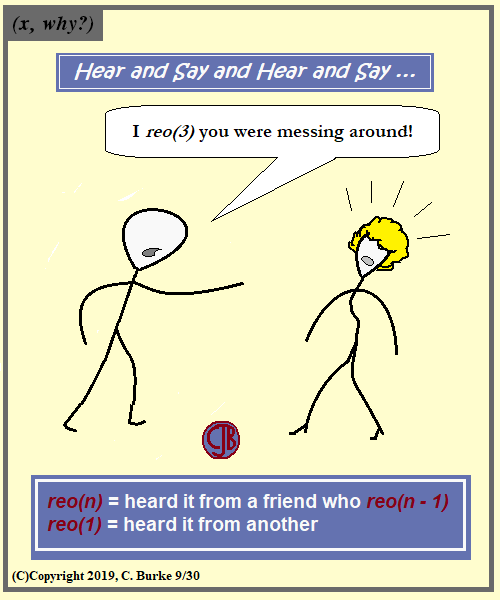Don't confuse this with the rem(n) function!

I did just see the band last week, so, yes, I was thinking about math and comics during a concert.

And the "rem()" function, whatever that is, will have to wait for another day. Not that I'm likely to see them in concert any time soon.

### Update: Bonus Comic

Hearsay being in the news right now, and my seeing REO Speedwagon in concert last week, several ideas came to mind, and I had to decide which one to use.
Here is another take on the song, more topical, but less mathematical.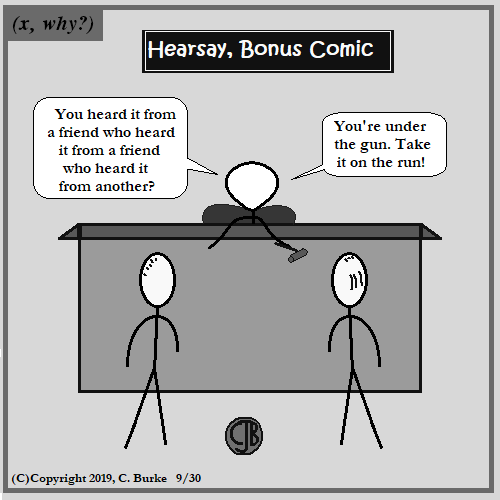Come back often for more funny math and geeky comics.## Friday, September 27, 2019

### Dividing 9's By 9

(Click on the comic if you can't see the full image.)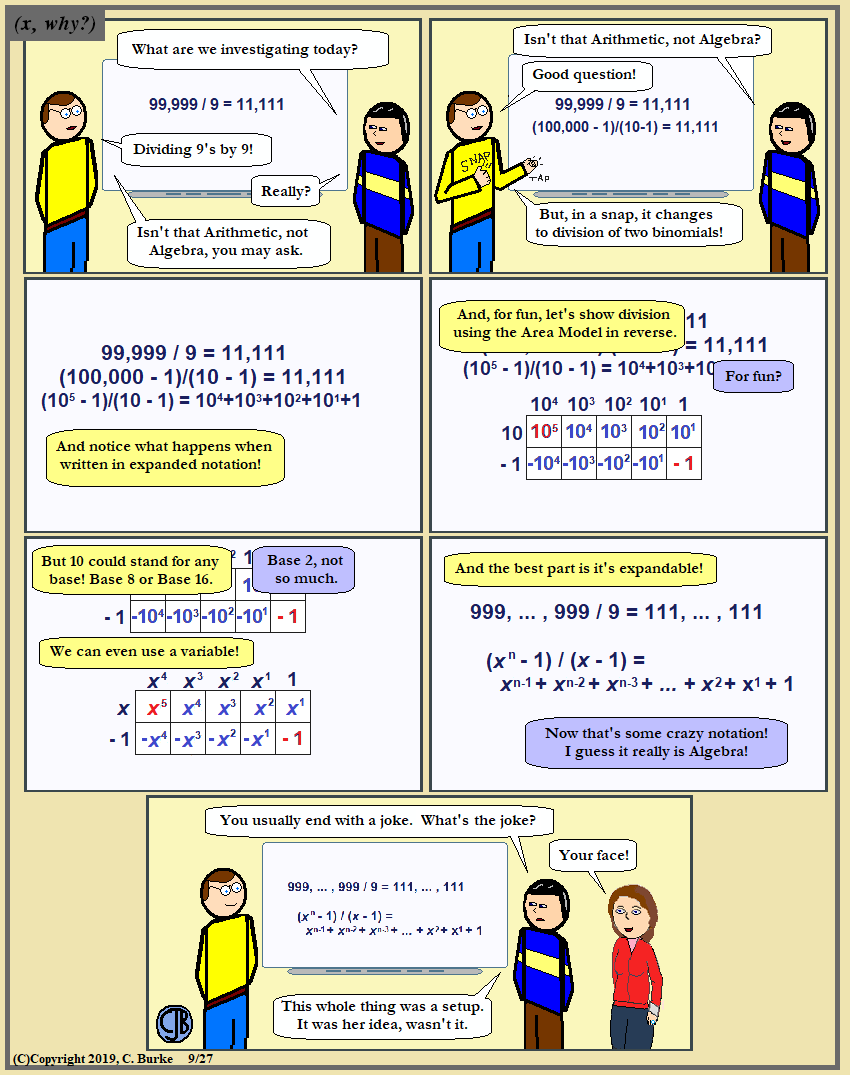And you can use x + 1 for 11's.

Instead of FOIL, I'm trying the "Box Method" again, only this time calling it The Area Model, and stressing the area of box, and the fact that it's a multiplication table. (I abandoned it years ago, because students would fill up tables and say, "I'm done!" without completing the problem. "That's not the answer. That's the work that gives you the answer.")

Using this in reverse, which in some cases is the same as Factoring By Grouping, the Area Model does division in a much nicer way than the traditional Long Division method, at least when there isn't a remainder. Actually, it's not that bad then, either.

Oh, and I have Snapped my fingers to distract from tapping the board to make the screen advance to the next slide. Sleight of hand is usually appreciated, even when they're on to you, if only because the other teachers aren't doing that.

Come back often for more funny math and geeky comics.## Thursday, September 26, 2019

### Hands

(Click on the comic if you can't see the full image.)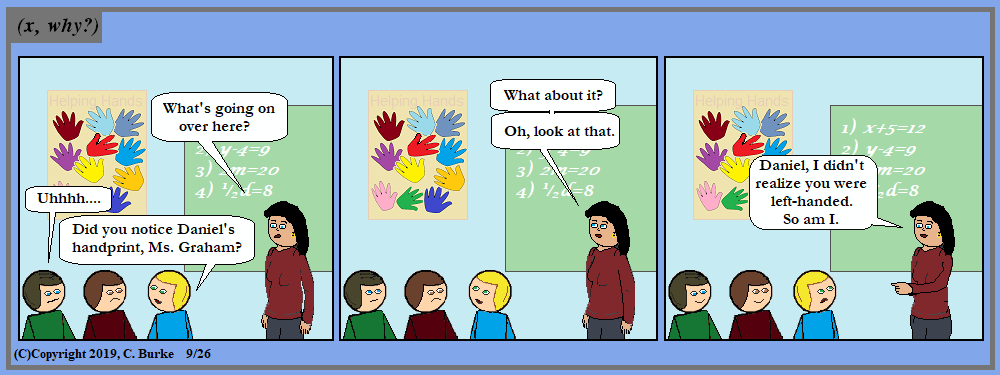That's what stands out the most, right?

If anyone is confused, they traced their hands, and cut them out.
The original activity would have included writing on the fingers, but that just too small to see.

New students here because I didn't want to use Ms. Graham's other students who we've seen in the past.

Come back often for more funny math and geeky comics.## Tuesday, September 24, 2019

### (x, why?) Mini: Heptagon

(Click on the comic if you can't see the full image.)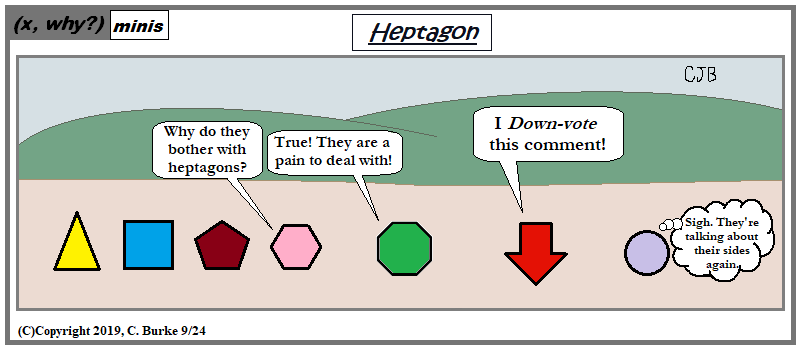Do sides matter? If we're talking a side of fries or a side of kale, then, yes, they certainly matter!

Don't downvote me!

Amusing aside: many of the houses I use for background are actually heptagons.

Come back often for more funny math and geeky comics.## Friday, September 20, 2019

### School Life #10: Girlfriend

(Click on the comic if you can't see the full image.)Students change. Group dynamics change. The cast changes, too, but often changes right back again.

School Life is a collection of strips about the students in school (or possibly outside of it) that don't fall into the usual scholastic-based humor. With enough backstory, I could write my own fanfic based on these guys. Granted, I'd have to give them all names first. Added one today. Up until now, I had the four new girls as Filipino (and loosely based on groups of students I knew), but the more I looked at Kyung, the more I thought that maybe she could be Korean (possibly North?) who is befriended by the other three.

So welcome to (temporarily non-canonical, until I say so) Kyung Mi Park. The other three girls have Spanish surnames that I haven't decided on (but I'm leaning toward some former coworkers to whom I owe a great deal), with the tall one possibly named "Katie". The other two may or may not have somewhat "saintly" names, or American variations thereof.

In the meantime, I'm still deciding on the stories to tell, how to tell them, and how much of them to tell, because it really isn't the main focus of the comic. And as much as I deal with these youngsters transforming into adults (I can't always call them "young adults" just yet), their stories aren't mine to tell. To adapt, maybe, in a way to bring them out to my readers, but not to tell outright.

There could be another ten of these before the year is out, or there might not even be ten more in the future. Time will tell.

But Daisy won't. She's good with secrets.

Come back often for more funny math and geeky comics.## Wednesday, September 18, 2019

### Pause

(Click on the comic if you can't see the full image.)It's a *pregnant* pause!

So this may go on a while, and we probably won't see the baby before next summer break ... unless I get desperate for a story line.

The bonus is that not only wouldn't a student teacher return again, unless they were in a time lock, but in the Ten Years comic, we learned that she was one of the comic's original students ... All Growed Up!

Come back often for more funny math and geeky comics.## Monday, September 16, 2019

### Bigons

(Click on the comic if you can't see the full image.)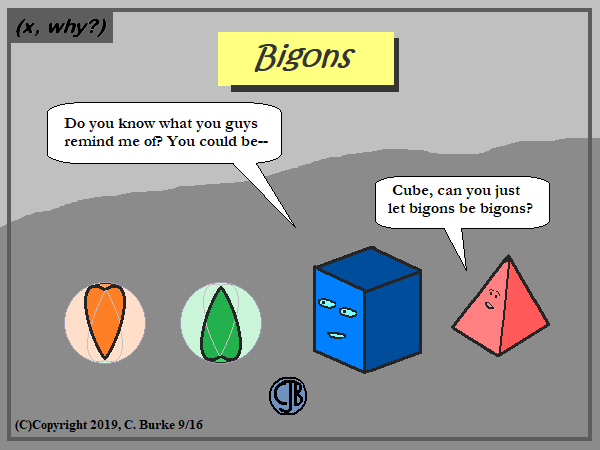Spherical lunes, to be exact.

Not the Cube. He's just a loon.

Come back often for more funny math and geeky comics.## Friday, September 13, 2019

### Friday Full Moon 13th

(Click on the comic if you can't see the full image.)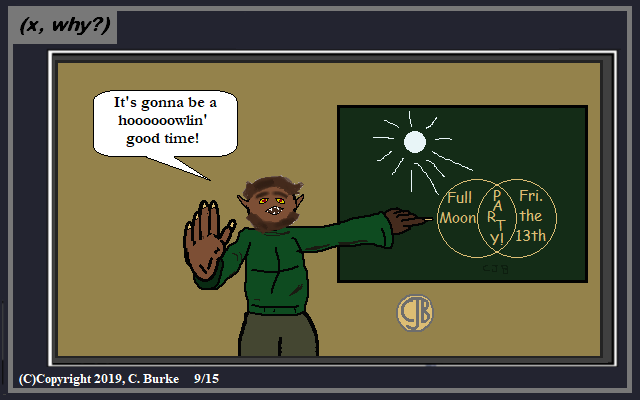You know he'll be vamping about, life of the party of the undead.

Complete on 9/15 for 9/13. So much for punctuality.

Come back often for more funny math and geeky comics.## Tuesday, September 10, 2019

### Palindrome Dates

(Click on the comic if you can't see the full image.)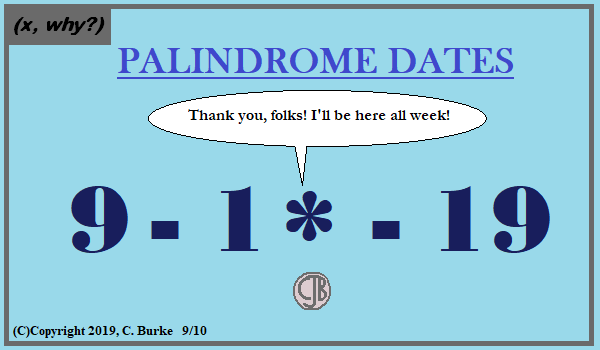I shy away from a lot of obvious date jokes, but it's the last palindrome week of the century, so I don't think I'll be doing it again!

Come back often for more funny math and geeky comics.## Friday, September 06, 2019

### Summer Expectations

(Click on the comic if you can't see the full image.)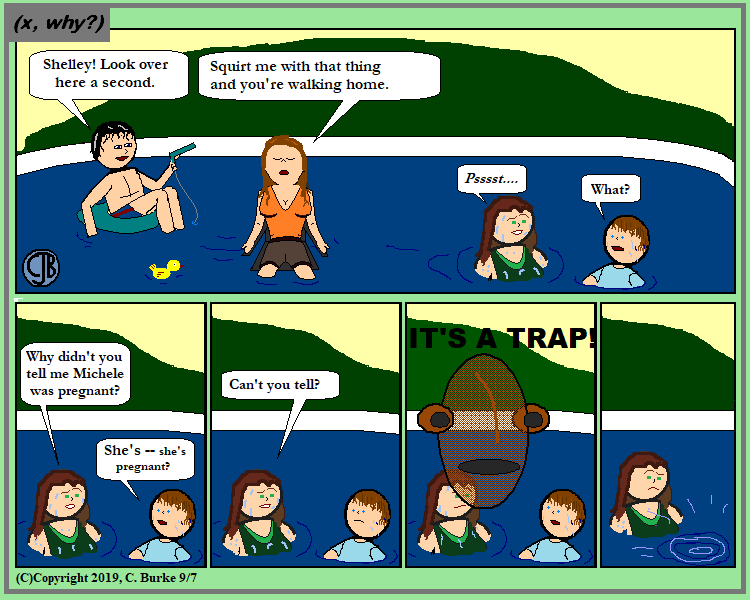I wanted a beat panel, followed by uncontrollable laughter, and then sliding under, but it was too space. So I went with Ackbar.

This image was created on Saturday 9/7 for Friday's strip. It was late, and I've fallen behind a bit.
Hopefully, Monday's strip will appear on Monday.

Actually, this would have been a good, ahem, Labor Day strip, if only because three of them went back to work right afterward.

Come back often for more funny math and geeky comics.## Wednesday, September 04, 2019

### Summer Travel

(Click on the comic if you can't see the full image.)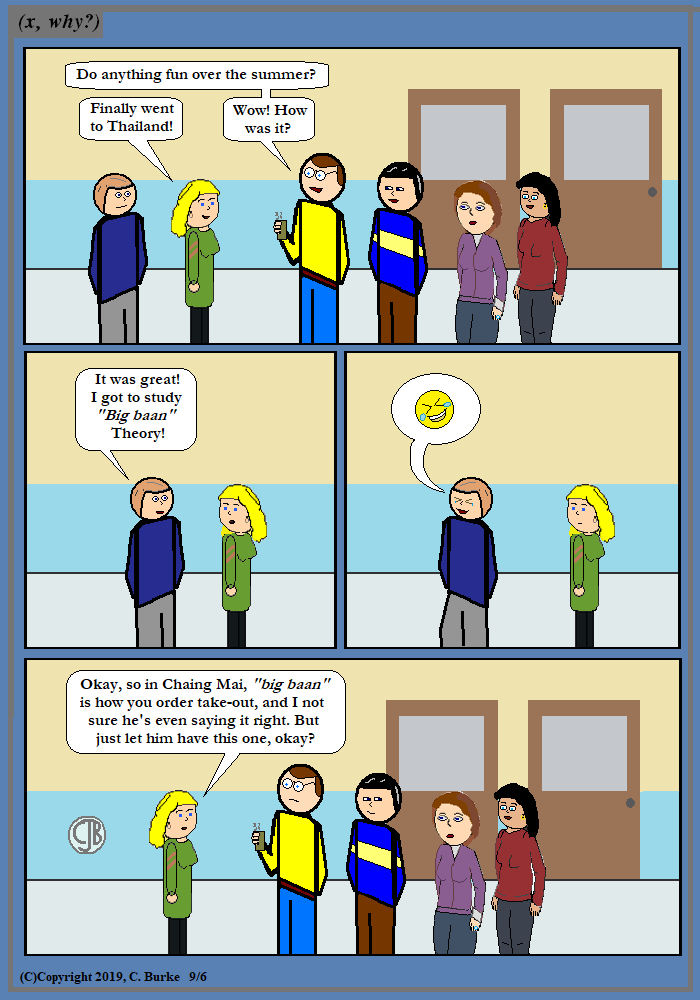I wanted to say it was a "Thai" for the best summer story, but I didn't have any others to run with.

This image was created on Friday 9/6 for Wednesday's strip. It was late, and I've fallen behind a bit.
Hopefully, Friday's strip will be created on Friday as well.

Come back often for more funny math and geeky comics.## Tuesday, September 03, 2019

### August 2019 Geometry Regents Part 1 (multiple choice)

The following are some of the multiple questions from the recent June 2019 New York State Common Core Geometry Regents exam.

### August 2019 Geometry, Part I

Each correct answer is worth up to 2 credits. No partial credit. Work need not be shown.

1. On the set of axes below, AB is dilated by a scale factor of 5/2 centered at point P.

Which statement is always true?

Shape (and therefore slope) is preserved in a dilation, so the line segments are parallel.
Choice (4) is backward. If it had been 5/2(AB) = A'B', that would have been correct.

2. The coordinates of the vertices of parallelogram CDEH are C(-5,5), D(2,5), E(-1,-1), and H(-8,-1). What are the coordinates of P, the point of intersection of diagonals CE and DH?

In a parallelogram, the diagonals bisect each other, so the point of intersection must be the midpoint of both lines.
The midpoint of CE is at ( (-5+-1)/2, (5+-1)/2 ), which is (-3, 2).
Checking the other line, just to be sure:
The midpoint of DH is at ( (2+-8)/2, (5+-1)/2 ), which is (-3, 2).

3. The coordinates of the endpoints of QS are Q(-9,8) and S(9,-4). Point R is on QS such that QR:RS is in the ratio of 1:2. What are the coordinates of point R?

A ratio of 1:2 means one-third of the way along he line.
The difference in the x-coordinates is 9 - (-9) = 18. One-third of 18 is 6, and -9 + 6 = -3.
The eliminates all but one choice.
Check the y-coordinates to be sure:
The difference in the y-coordinates is -4 - 8 = -12. One-third of -12 is -4, and 8 + -4 = 4.

4. If the altitudes of a triangle meet at one of the triangle’s vertices, then the triangle is

There's no way for this to happen unless one of the angles is a right angle, which makes it a right triangle.

5. In the diagram below of triangle ACD, DB is a median to AC, and AB = DB

If m∠DAB = 32°, what is m∠BDC?

If m∠DAB = 32°, then m∠ADB = 32° because it is an isosceles triangle.
This makes m∠DBC = 64° by the Remote Angle Theorem.
Since B is a median, AB = BC, but since AB = DB, then BC = DB, making DBC an isosceles triangle.
This makes BDC = BCD, and the pair of base angles have a sum equal to 180 - 64 = 116.
Half of 116 is 58, so m∠BDC = 58°

6. What are the coordinates of the center and the length of the radius of the circle whose equation is x2 + y2 = 8x - 6y + 39?

To put is into standard form (x - h)2 + (y - k)2 = r2, you need to move the x and y terms to the left side and then Complete the Square, twice.
Remember, you need to take half of the coefficient of x (or y) and then square it to find out what to add.

x2 + y2 = 8x - 6y + 39
x2 - 8x + y2 + 6y = 39
x2 - 8x + 16 + y2 + 6y + 9 = 39 + 16 + 9
(x - 4)2 + (y + 3)2 = 64

Quick answer: Move the x and y term to the left, you get -8x and +6y.
This tells you that the center is going to (-1/2) of those coefficients: (4, -3)
The radius is the square root of the number on the right, and 64^2 is a really big number, so it doesn't make sense.

7. In the diagram below of parallelogram ABCD, AFGB, CF bisects <DCB, DG bisects &ADC, and CF and DG intersect at E.

If m<B = 75o, then the measure of <EFA is

The opposite angles of a parallelogram are congruent, and consecutive angles are supplementary. Both C and D are bisected, which cuts them in half. Since the sides are parallel, DG and CF are transversals and the alternate interior angles will be congruent.
Label the angles you know until you get to the one that you want.

Angle B is 75, so Angle DCB is 105, which is split into 52.5 and 52.5.
Alternate interior angles tells us that CFG is also 52.5. This makes Angle EFA 127.5°.

8. What is an equation of a line that is perpendicular to the line whose equation is 2y + 3x = 1?

Answer: (1) y = 2/3 x + 5/2
If you convert to slope-intercept form, you get
2y = -3x + 1
y = -3/2 x + 1/2
This is the same line, not the line perpendicular to it, which will have a slope that is the multiplicative inverse of -3/2, which is 2/3.

In this problem, the y-intercept is not important because you aren't given a specific point, and only one choice has the slope 2/3.

9. Triangles ABC and RST are graphed on the set of axes below.

Which sequence of rigid motions will prove triagnle ABC = triangle RST?

Answer: (2) a rotation of 180° centered at (1,0)
Notice the orientation of the two triangles.
A line reflection over y = x would move A to (7, -1), not to point R.
A line reflection over the x-axis would produce the mirror image of RST.
A line reflection over the x-axis followed by a line reflection over y = 1 looks okay -- until you realize that you need to reflect over x = 1 not y. Don't fall for this one.

10. If the line represented by y = - 1/4 x - 2 is dilated by a scale factor of 4 centered at the origin, which statement about the image is true?

Answer: (1) The slope is - 1/4 and the y-intercept is -8.
The slope of the line would not change. The y-intercept would move four times the distance from the origin, so -2 becomes -8.

11. Square MATH has a side length of 7 inches. Which three-dimensional object will be formed by continuously rotating square MATH around side AT?

A square would become a cylinder in three dimensions. If it is rotated about one of its sides, then the side length would become the radius.

12. Circle O with a radius of 9 is drawn below. The measure of central angle AOC is 120°.
What is the area of the shaded sector of circle O?

The shaded area is 240 degrees, or 2/3 of the area of the entire circle.
A = (2/3) (pi) (9)2 = 54 pi

13. In quadrilateral QRST, diagonals QS and RT intersect at M. Which statement would always prove quadrilateral QRST is a parallelogram?

Answer: (3) QR = TS and QT = RS
If the opposite sides of a quadrilateral are congruent, the figure is a parallelogram.
The others could be true for some trapezoids.

14. A standard-size golf ball has a diameter of 1.680 inches. The material used to make the golf ball weighs 0.6523 ounce per cubic inch. What is the weight, to the nearest hundredth of an ounce, of one golf ball?

Find the volume is cubic inches. Then multiply be the weight per cubic inch.
Note that you were given Diameter, and not Radius.
V = (4/3) (pi) (r)3 = (4/3)*(3.141592)(1.680/2)3 = 2.48...
Weight = (2.48)(0.6523) = 1.617 = 1.62

15. Chelsea is sitting 8 feet from the foot of a tree. From where she is sitting, the angle of elevation of her line of sight to the top of the tree is 36°. If her line of sight starts 1.5 feet above ground, how tall is the tree, to the nearest foot?

The distance to the tree is adjacent. The height of the tree is opposite. That means use tangent.
Tan 36 = x / 8
x = 8 * tan 36 = 5.81234...
Add 1.5 for her height while sitting: 1.5 + 5.8 = 7.3

16. In the diagram below of right triangle ABC, altitude CD intersects hypotenuse AB at D.

Which equation is always true?

Short leg is to hypotenuse as short leg is to hypotenuse.
The corresponding sides are proportionals of the two smaller right triangles and the larger one.

17. A countertop for a kitchen is modeled with the dimensions shown below. An 18-inch by 21-inch rectangle will be removed for the installation of the sink.

What is the area of the top of the installed countertop, to the nearest square foot?

Cut it into parts: rectangle 1 + rectangle 2 - rectangle 3
2 * 8 + (5-2) * 2 - (18/12 * 21/12) = 19.375 square feet

18. In the diagram below, BC connects points B and C on the congruent sides of isosceles triangle ADE, such that triangle ABC is isosceles with vertex angle A. If AB = 10, BD = 5, and DE = 12, what is the length of BC?

Corresponding sides are proportional. Just remember that you need to use AD, not BD
10/15 = BC/12
15 BC = 120
BC = 8

Also, 10/15 = 2/3, and 2/3 of 12 is 8.

19. In triangle ABC below, angle C is a right angle.
[Image Omitted]
Which statement must be true?

Answer: (1) sin A = cos B
What is opposite of angle A is adjacent to angle B.
Choice (4) is only true if A and B are both 45 degrees.

20. In right triangle RST, altitude TV is drawn to hypotenuse RS. If RV = 12 and RT = 18, what is the length of SV?

If you sketch the figure, you will see that RT is the base of a small right triangle and RT is its hypotenuse. In the larger triangle, RST, RT is the base, and RS is the hypotenuse. So you can set up a proportion:
12 / 18 = 18 / RS
12 RS = 18 * 18
RS = 18 * 18 / 12 = 27
(Also, 12/18 reduces to 2/3, and 18 is 2/3 of 27.)

Finally, SV = RS - RV = 27 - 12 = 15.

21. What is the volume, in cubic centimeters, of a right square pyramid with base edges that are 64 cm long and a slant height of 40 cm

You need the height of the pyramid, not the slant height. Use the Pythagorean Theorem with the slant height as the hypotenuse and half of the side length as the base. (Use half because you only want to get to the center.)
Note: Check to see if it's a multiple of 3-4-5 to save yourself a bunch of work.
If not, or if you aren't sure, continue:

322 + h2 = 402
1024 + h2 = 1600
h2 = 576
h = 24

Volume = (1/3) Area of base * height
V = (1/3) * 642 * 24 = 32,768

Note 1: 24 - 32 - 40 is a multiple of 3 - 4 - 5.
Note 2: Choice (4) 54613.3... is the result if you used the slant height instead of the height. Offhand, I don't know what mistakes might lead you to getting choices (1) and (2).

22. In the diagram below, chords PQ and RS of circle O intersect at T.
[Image omitted]
Which relationship must always be true?

Answer: (4) RT X TS = PT X TQ
When two chords intersect each other, the product of their segments will be equal.
The sums (Choice (3)) usually will not be because chords of a circle can be any size, up to the length of the diameter.

23. A rhombus is graphed on the set of axes below.
[IMAGE OMITTED]
Which transformation would carry the rhombus onto itself?

Answer: (4) A reflection over the line x = 0
Hopefully, it is obvious that the y-axis divides the rhombus in half. So reflecting over the y-axis will carry it onto itself. That is, if A were (-4, 1) and C were (4, 1), then A' = C and C' = A. The other two vertices would carry onto themselves (they don't move).

The y-axis has the equation x = 0.
The line y = 0 is the x-axis, which does not divide the rhombus into two congruent figures.
Rotating around the origin would leave the image mostly below the x-axis instead of above.
Reflecting over y = 1/2x + 1 would have the rhombus stretched up and down, instead of side to side. That is, the longer diagonal would be vertical, not horizontal.

24. A 15-foot ladder leans against a wall and makes an angle of 65o with the ground. What is the horizontal distance from the wall to the base of the ladder, to the nearest tenth of a foot?